• 支持向量机（support vector machines, SVM）是一种二分类模型，它的基本模型是定义在特征空间上的间隔最大的线性分类器，间隔最大使它有别于感知；SVM还包括核技巧，这使它成为实质上的非线性分类器。SVM的的学习...
• 线性回归（一）、逻辑回归（二）、K近邻（三）、决策树值ID3（四）、CART（五）、感知（六）、神经网络（七）、线性可分支持向量机（八）、线性支持向量机（九）、线性不可分支持向量机（十）、朴素贝叶斯（十一）...
• SVM.py,支持向量机python 代码实现
• SVM支持向量机Python实现，大家可以当备用资料存储，也可以直接实际操作SMO SVM
• 主要介绍了Python中的支持向量机SVM的使用（附实例代码），文中通过示例代码介绍的非常详细，对大家的学习或者工作具有一定的参考学习价值，需要的朋友们下面随着小编来一起学习学习吧
• 在机器学习领域，支持向量机SVM(Support Vector Machine)是一个有监督的学习模型，通常用来进行模式识别、分类(异常值检测)以及回归分析。 其具有以下特征：  (1)SVM可以表示为凸优化问题，因此可以利用已知的...
• ∗∗∗ 点击查看 :吴恩达...∗∗∗ ...本次作业的理论部分：吴恩达机器学习（七）支持向量机 编程环境：Jupyter Notebook 1. 线性 SVM 任务 观察惩罚项系数 C 对决策边界的影响，数据集：data/ex6data1.mat 在理论部分，
• python利用支持向量机SVM进行时间序列预测, 包括数据和python代码 python利用支持向量机SVM进行时间序列预测, 包括数据和python代码
• 用于支持向量机分类，内置网格搜索算法与K折交叉验证，给出最佳分类参数。
• 主要介绍了Python SVM(支持向量机)实现方法,结合完整实例形式分析了基于Python实现向量SVM算法的具体步骤与相关操作注意事项,需要的朋友可以参考下
• 支持向量机SVM python源代码 亲测可在pycharm用，可以用于统计学习方法的课后练习使用
• SVM支持向量机Python代码，更改训练数据集和测试数据集可用
• 以分类精度为目标的传统分类算法通常假定:每个样本的误分类具有同样...针对此缺隙,并基于标准的SVM,通过在SVM的设计中集成样本的不同误分类代价,提出代价敏感支持向量机(CS-SVM)的设计方法.实验结果表明CS-SVM是有效的.
• python用SVM实现手写数字识别 import matplotlib.pyplot as plt from sklearn import datasets, svm, metrics #载入数据集 digits = datasets.load_digits() # The data that we are interested in is made of 8x8 ...
python用SVM实现手写数字识别
import matplotlib.pyplot as plt
from sklearn import datasets, svm, metrics
#载入数据集

# The data that we are interested in is made of 8x8 images of digits, let's
# have a look at the first 4 images, stored in the images attribute of the
# dataset.  If we were working from image files, we could load them using
# matplotlib.pyplot.imread.  Note that each image must have the same size. For these
# images, we know which digit they represent: it is given in the 'target' of
# the dataset.
images_and_labels = list(zip(digits.images, digits.target))
#画图
for index, (image, label) in enumerate(images_and_labels[:4]):
plt.subplot(2, 4, index + 1)
plt.axis('off')
plt.imshow(image, cmap=plt.cm.gray_r, interpolation='nearest')
plt.title('Training: %i' % label)

#取图片样本数
n_samples = len(digits.images)
#降维
data = digits.images.reshape((n_samples, -1))
#调用svm算法，其中gamma=0.001
classifier = svm.SVC(gamma=0.001)
#拟合
classifier.fit(data[:n_samples // 2], digits.target[:n_samples // 2])

#测试集数据
expected = digits.target[n_samples // 2:]
#预测
predicted = classifier.predict(data[n_samples // 2:])

print("Classification report for classifier %s:\n%s\n"
% (classifier, metrics.classification_report(expected, predicted)))
print("Confusion matrix:\n%s" % metrics.confusion_matrix(expected, predicted))

images_and_predictions = list(zip(digits.images[n_samples // 2:], predicted))
#画图
for index, (image, prediction) in enumerate(images_and_predictions[:4]):
plt.subplot(2, 4, index + 5)
plt.axis('off')
plt.imshow(image, cmap=plt.cm.gray_r, interpolation='nearest')
plt.title('Prediction: %i' % prediction)
plt.show()

运行结果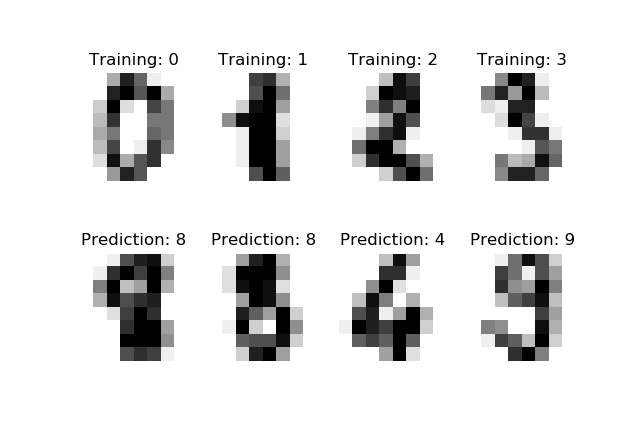展开全文机器学习
• 使用python手动实现了SVM支持向量机，包括其中二次规划的求解（调用cvxopt包），实现了软间隔及核技术，以及对数据集及分类效果的可视化！建议配合我的SVM PPT一起学习SVM 不是直接调用sklearn的SVM！！
• 参见：https://blog.csdn.net/qq_41577045/article/details/79859924 向大神致敬！！！ 代码1： #matplotlib inline import matplotlib.pyplot as plt ...class1 = np.array([[1, 1], [1, 3], [2, 1], [1, 2]...
参见：https://blog.csdn.net/qq_41577045/article/details/79859924
向大神致敬！！！
代码1：
#matplotlib inline
import matplotlib.pyplot as plt
import numpy as np

class1 = np.array([[1, 1], [1, 3], [2, 1], [1, 2], [2, 2]])
class2 = np.array([[4, 4], [5, 5], [5, 4], [5, 3], [4, 5], [6, 4]])

plt.figure(figsize=(6, 4), dpi=120)

plt.title('Decision Boundary')

plt.xlim(0, 8)
plt.ylim(0, 6)
ax = plt.gca()                                  # gca 代表当前坐标轴，即 'get current axis'
ax.spines['right'].set_color('none')            # 隐藏坐标轴
ax.spines['top'].set_color('none')

plt.scatter(class1[:, 0], class1[:, 1], marker='o')
plt.scatter(class2[:, 0], class2[:, 1], marker='s')
plt.plot([1, 5], [5, 1], '-r')
plt.arrow(4, 4, -1, -1, shape='full', color='r')
plt.plot([3, 3], [0.5, 6], '--b')
plt.arrow(4, 4, -1, 0, shape='full', color='b', linestyle='--')
plt.annotate(r'margin 1',
xy=(3.5, 4), xycoords='data',
xytext=(3.1, 4.5), fontsize=10,
plt.annotate(r'margin 2',
xy=(3.5, 3.5), xycoords='data',
xytext=(4, 3.5), fontsize=10,
plt.annotate(r'support vector',
xy=(4, 4), xycoords='data',
xytext=(5, 4.5), fontsize=10,
plt.annotate(r'support vector',
xy=(2, 2), xycoords='data',
xytext=(0.5, 1.5), fontsize=10,

plt.savefig("SVM1.jpg")

plt.show()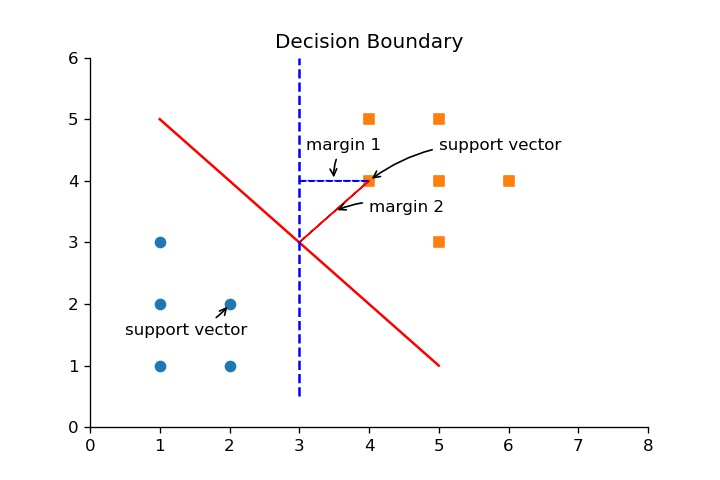代码2：
#matplotlib inline
import matplotlib.pyplot as plt
import numpy as np

class1 = np.array([[1, 1], [1, 3], [2, 1], [1, 2], [2, 2]])
class2 = np.array([[4, 4], [5, 5], [5, 4], [5, 3], [4, 5], [6, 4]])

plt.figure(figsize=(6, 4), dpi=120)

plt.title('Support Vector Machine')

plt.xlim(0, 8)
plt.ylim(0, 6)
ax = plt.gca()                                  # gca 代表当前坐标轴，即 'get current axis'
ax.spines['right'].set_color('none')            # 隐藏坐标轴
ax.spines['top'].set_color('none')

plt.scatter(class1[:, 0], class1[:, 1], marker='o')
plt.scatter(class2[:, 0], class2[:, 1], marker='s')
plt.plot([1, 5], [5, 1], '-r')
plt.plot([0, 4], [4, 0], '--b', [2, 6], [6, 2], '--b')
plt.arrow(4, 4, -1, -1, shape='full', color='b')
plt.annotate(r'$w^T x + b = 0$',
xy=(5, 1), xycoords='data',
xytext=(6, 1), fontsize=10,
plt.annotate(r'$w^T x + b = 1$',
xy=(6, 2), xycoords='data',
xytext=(7, 2), fontsize=10,
plt.annotate(r'$w^T x + b = -1$',
xy=(3.5, 0.5), xycoords='data',
xytext=(4.5, 0.2), fontsize=10,
plt.annotate(r'd',
xy=(3.5, 3.5), xycoords='data',
xytext=(2, 4.5), fontsize=10,
plt.annotate(r'A',
xy=(4, 4), xycoords='data',
xytext=(5, 4.5), fontsize=10,

plt.savefig("SVM2.jpg")

plt.show()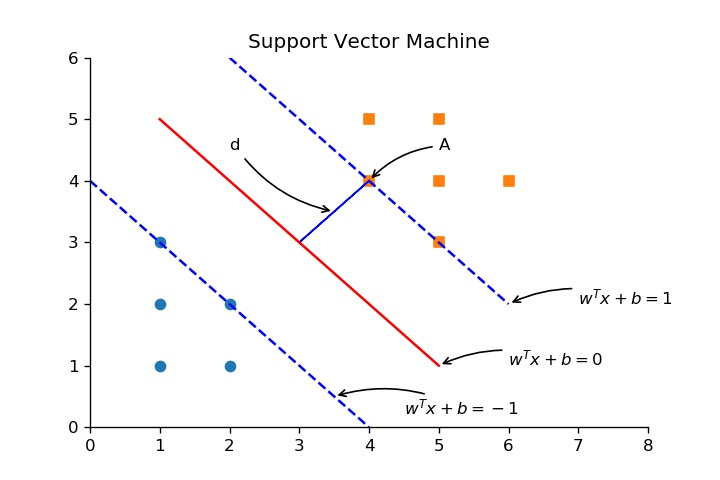代码3：
#matplotlib inline
import matplotlib.pyplot as plt
import numpy as np

from sklearn.datasets import make_blobs

plt.figure(figsize=(10, 4), dpi=140)

# sub plot 1
plt.subplot(1, 2, 1)

X, y = make_blobs(n_samples=100,
n_features=2,
centers=[(1, 1), (2, 2)],
random_state=4,
shuffle=False,
cluster_std=0.4)

plt.title('Non-linear Separatable')

plt.xlim(0, 3)
plt.ylim(0, 3)
ax = plt.gca()                                  # gca 代表当前坐标轴，即 'get current axis'
ax.spines['right'].set_color('none')            # 隐藏坐标轴
ax.spines['top'].set_color('none')

plt.scatter(X[y==0][:, 0], X[y==0][:, 1], marker='o')
plt.scatter(X[y==1][:, 0], X[y==1][:, 1], marker='s')
plt.plot([0.5, 2.5], [2.5, 0.5], '-r')

# sub plot 2
plt.subplot(1, 2, 2)

class1 = np.array([[1, 1], [1, 3], [2, 1], [1, 2], [2, 2], [1.5, 1.5], [1.2, 1.7]])
class2 = np.array([[4, 4], [5, 5], [5, 4], [5, 3], [4, 5], [6, 4], [5.5, 3.5], [4.5, 4.5], [2, 1.5]])

plt.title('Slack Variable')

plt.xlim(0, 7)
plt.ylim(0, 7)
ax = plt.gca()                                  # gca 代表当前坐标轴，即 'get current axis'
ax.spines['right'].set_color('none')            # 隐藏坐标轴
ax.spines['top'].set_color('none')

plt.scatter(class1[:, 0], class1[:, 1], marker='o')
plt.scatter(class2[:, 0], class2[:, 1], marker='s')
plt.plot([1, 5], [5, 1], '-r')
plt.plot([0, 4], [4, 0], '--b', [2, 6], [6, 2], '--b')
plt.arrow(2, 1.5, 2.25, 2.25, shape='full', color='b')
plt.annotate(r'violate margin rule.',
xy=(2, 1.5), xycoords='data',
xytext=(0.2, 0.5), fontsize=10,
plt.annotate(r'normal sample. $\epsilon = 0$',
xy=(4, 5), xycoords='data',
xytext=(4.5, 5.5), fontsize=10,
plt.annotate(r'$\epsilon > 0$',
xy=(3, 2.5), xycoords='data',
xytext=(3, 1.5), fontsize=10,

plt.savefig("SVM3.jpg")

plt.show()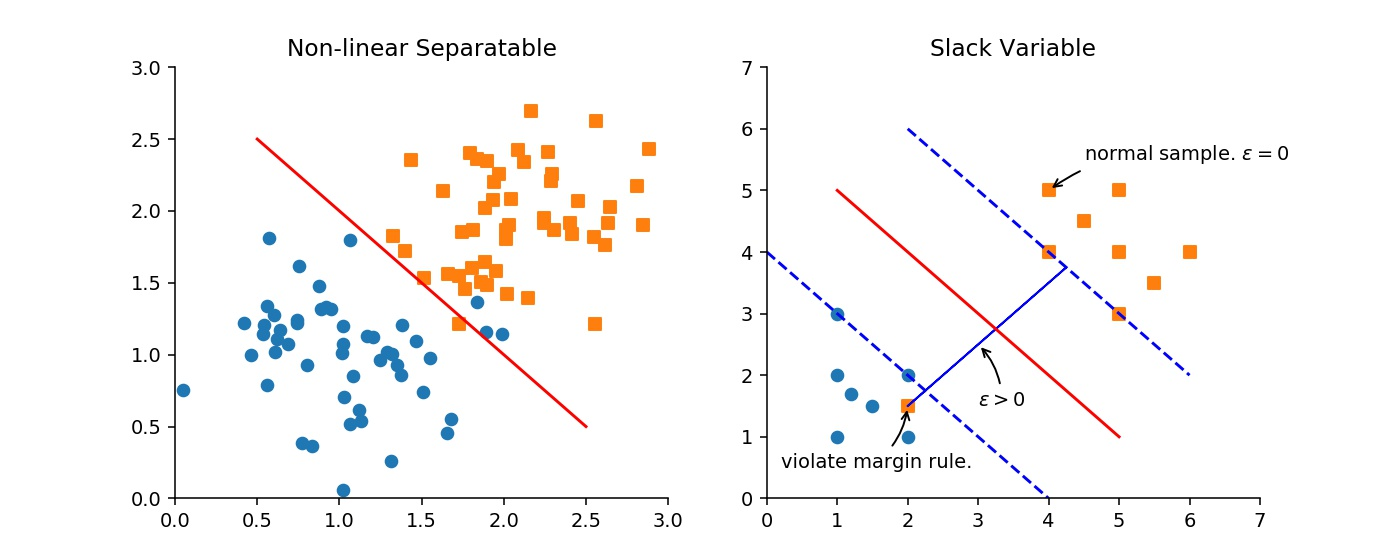代码4：
#matplotlib inline
import matplotlib.pyplot as plt
import numpy as np

plt.figure(figsize=(6, 4), dpi=120)

plt.title('Cost')

plt.xlim(0, 4)
plt.ylim(0, 2)
plt.xlabel('$y^{(i)} (w^T x^{(i)} + b)$')
plt.ylabel('Cost')
ax = plt.gca()                                  # gca 代表当前坐标轴，即 'get current axis'
ax.spines['right'].set_color('none')            # 隐藏坐标轴
ax.spines['top'].set_color('none')

plt.plot([0, 1], [1.5, 0], '-r')
plt.plot([1, 3], [0.015, 0.015], '-r')
plt.annotate(r'$J_i = R \epsilon_i$ for $y^{(i)} (w^T x^{(i)} + b) \geq 1 - \epsilon_i$',
xy=(0.7, 0.5), xycoords='data',
xytext=(1, 1), fontsize=10,
plt.annotate(r'$J_i = 0$ for $y^{(i)} (w^T x^{(i)} + b) \geq 1$',
xy=(1.5, 0), xycoords='data',
xytext=(1.8, 0.2), fontsize=10,

plt.savefig("SVM4.jpg")

plt.show()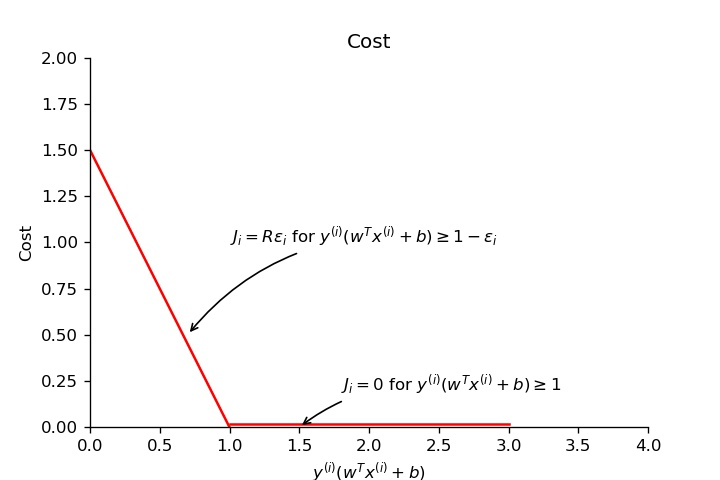代码5：
#matplotlib inline
import matplotlib.pyplot as plt
import numpy as np

plt.figure(figsize=(10, 4), dpi=144)

class1 = np.array([[1, 1], [1, 2], [1, 3], [2, 1], [2, 2], [3, 2], [4, 1], [5, 1]])
class2 = np.array([[2.2, 4], [1.5, 5], [1.8, 4.6], [2.4, 5], [3.2, 5], [3.7, 4], [4.5, 4.5], [5.4, 3]])

# sub plot 1
plt.subplot(1, 2, 1)

plt.title('Non-linear Separatable in Low Dimension')

plt.xlim(0, 6)
plt.ylim(0, 6)
plt.yticks(())
plt.xlabel('X1')
ax = plt.gca()                                  # gca 代表当前坐标轴，即 'get current axis'
ax.spines['right'].set_color('none')            # 隐藏坐标轴
ax.spines['top'].set_color('none')
ax.spines['left'].set_color('none')

plt.scatter(class1[:, 0], np.zeros(class1[:, 0].shape) + 0.05, marker='o')
plt.scatter(class2[:, 0], np.zeros(class2[:, 0].shape) + 0.05, marker='s')

# sub plot 2
plt.subplot(1, 2, 2)

plt.title('Linear Separatable in High Dimension')

plt.xlim(0, 6)
plt.ylim(0, 6)
plt.xlabel('X1')
plt.ylabel('X2')
ax = plt.gca()                                  # gca 代表当前坐标轴，即 'get current axis'
ax.spines['right'].set_color('none')            # 隐藏坐标轴
ax.spines['top'].set_color('none')

plt.scatter(class1[:, 0], class1[:, 1], marker='o')
plt.scatter(class2[:, 0], class2[:, 1], marker='s')
plt.plot([1, 5], [3.8, 2], '-r')

plt.savefig("SVM5.jpg")

plt.show()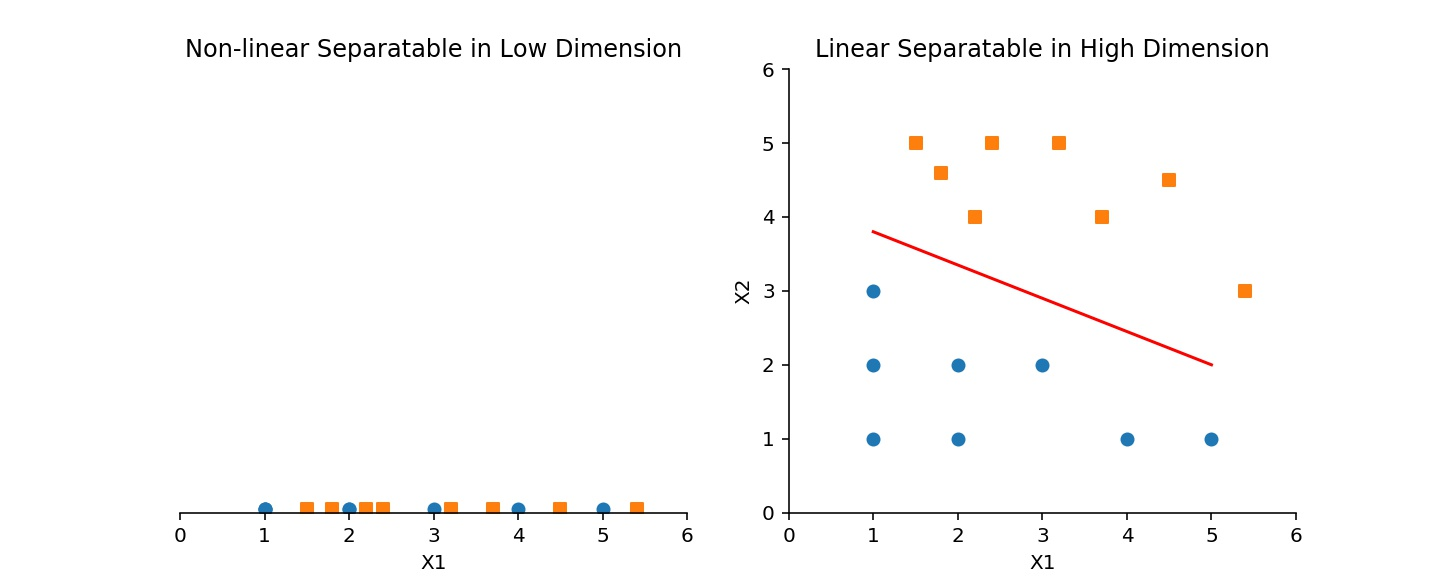代码6：
#matplotlib inline
import matplotlib.pyplot as plt
import numpy as np

def gaussian_kernel(x, mean, sigma):
return np.exp(- (x - mean)**2 / (2 * sigma**2))

x = np.linspace(0, 6, 500)
mean = 1
sigma1 = 0.1
sigma2 = 0.3

plt.figure(figsize=(10, 3), dpi=144)

# sub plot 1
plt.subplot(1, 2, 1)
plt.title('Gaussian for $\sigma={0}$'.format(sigma1))

plt.xlim(0, 2)
plt.ylim(0, 1.1)
ax = plt.gca()                                  # gca 代表当前坐标轴，即 'get current axis'
ax.spines['right'].set_color('none')            # 隐藏坐标轴
ax.spines['top'].set_color('none')

plt.plot(x, gaussian_kernel(x, mean, sigma1), 'r-')

# sub plot 2
plt.subplot(1, 2, 2)
plt.title('Gaussian for $\sigma={0}$'.format(sigma2))

plt.xlim(0, 2)
plt.ylim(0, 1.1)
ax = plt.gca()                                  # gca 代表当前坐标轴，即 'get current axis'
ax.spines['right'].set_color('none')            # 隐藏坐标轴
ax.spines['top'].set_color('none')

plt.plot(x, gaussian_kernel(x, mean, sigma2), 'r-')

plt.savefig("SVM6.jpg")

plt.show()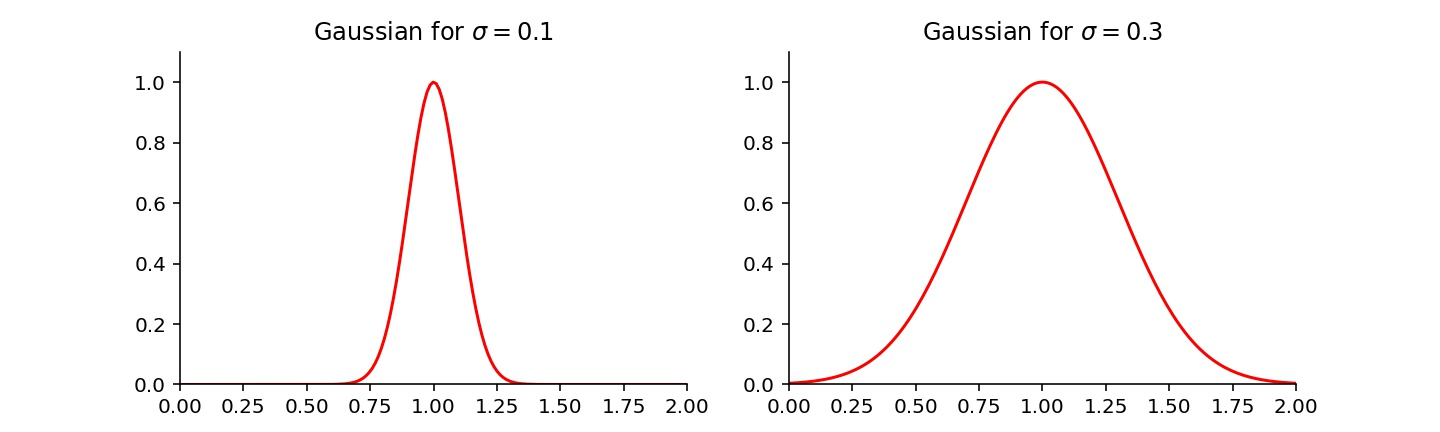欢迎关注微信公众号“源起1024”或扫描下方二维码获取更多学习资源展开全文• 经典书籍《统计学习方法》李航，第7章 支持向量机（SVM）-Python代码
• 最小二乘支持向量机（LSSVMtoolbox）--工具包
• Java、python、matlab三种语言实现svm算法，可直接运行查看结果。代码
• 主要介绍了python 机器学习之支持向量机非线性回归SVR模型，文中通过示例代码介绍的非常详细，对大家的学习或者工作具有一定的参考学习价值，需要的朋友们下面随着小编来一起学习学习吧
• 这是用粒子群优化支持向量机的小程序，数据是辛烷值的数据
• 支持向量机（Support Vector Machine, SVM）是一类按监督学习（supervised learning）方式对数据进行二元分类的广义线性分类器（generalized linear classifier），其决策边界是对学习样本求解的最大边距超平面...
• 本代码主要利用Python工具实现支持向量机，简单明了，易于理解
• 半监督支持向量机S3VM：半监督支持向量机（S3VM）方法HTML
• 支持向量机是一种在统计学习理论的基础上发展而来的机器学习方法，通过学习类别之间分界面附近的精确信息,可以自动寻找那些对分类有较好区分能力的支持向量,由此构造出的分类器可以使类与类之间的间隔最大化,因而...自动测试系统
• 支持向量机，是属于统计学习中的一种常见算法，但这种算法如果使用计算器计算的话也是浪费时间，哪怕是用计算机计算，也会很麻烦，所以使用代码来进行计算，简单快捷。 要实现的功能也很简单，我们传入一个文件，...
学习目标：
Python代码实现向量机

学习内容：
支持向量机，是属于统计学习中的一种常见算法，但这种算法如果使用计算器计算的话也是浪费时间，哪怕是用计算机计算，也会很麻烦，所以使用代码来进行计算，简单快捷。 要实现的功能也很简单，我们传入一个文件，文件中写有相关数据，然后代码自动计算w和b在，以及其它的一些参数，如下图，是我们的实现结果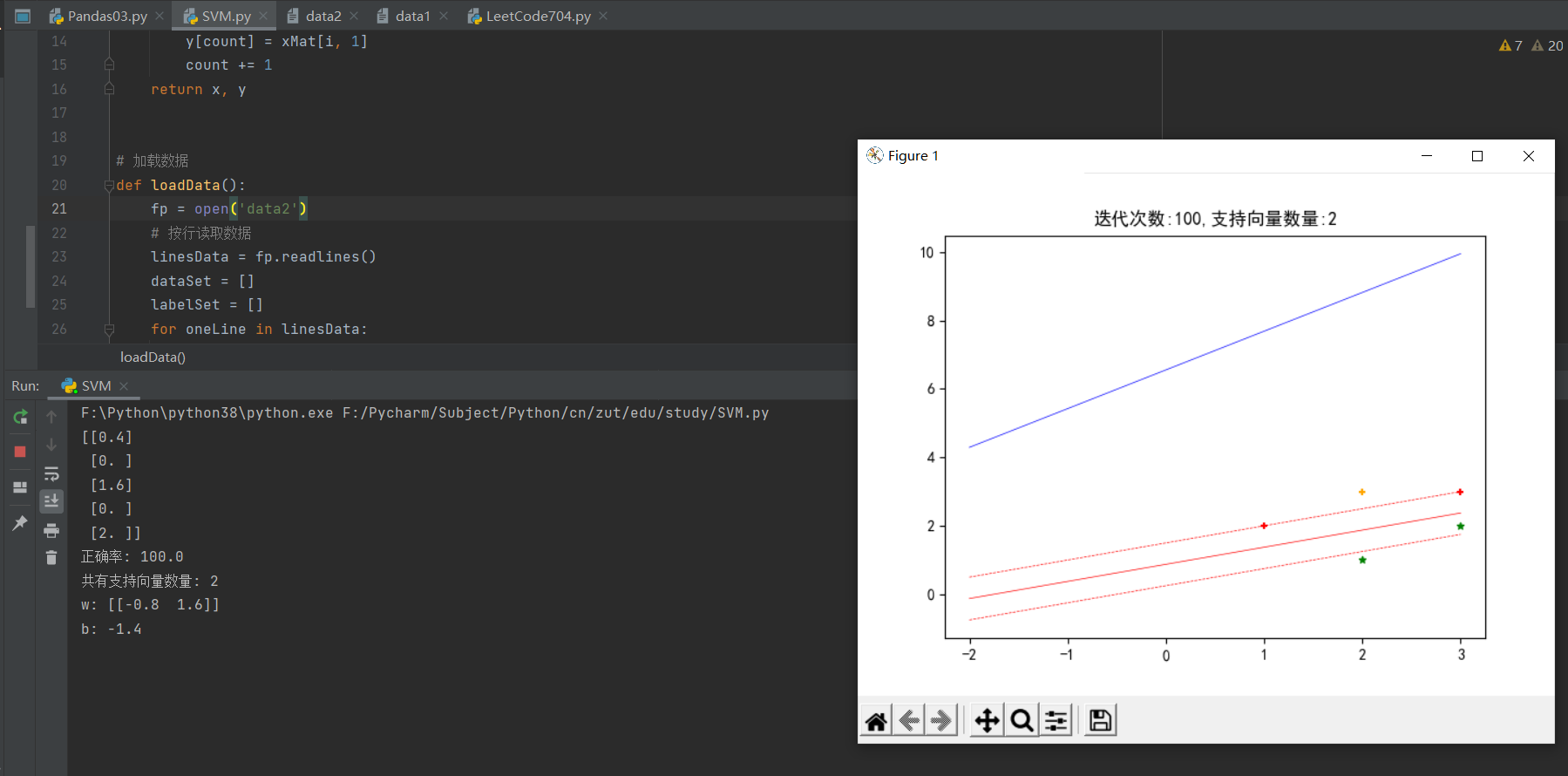代码实现：
直接上代码吧
from numpy import *
import matplotlib.pyplot as plt
from sklearn import svm

def getDataArray(lists, xMat):
x = np.ndarray(len(lists))
y = np.ndarray(len(lists))
count = 0
for i in lists:
x[count] = xMat[i, 0]
y[count] = xMat[i, 1]
count += 1
return x, y

# 加载数据
fp = open('data2')
# 按行读取数据
dataSet = []
labelSet = []
for oneLine in linesData:
oneLine = oneLine.split(',')
dataSet.append([float(oneLine.strip()), float(oneLine.strip())])
labelSet.append(float(oneLine.strip()))
return dataSet, labelSet

class SVM:
def __init__(self, xSet, yArray, C=None, floatingPointError=0.0001):
self.xMat = np.mat(xSet)  # (48,2)
self.yMat = np.mat(yArray).T  # (48,1)
self.rows = self.xMat.shape
self.cols = self.xMat.shape
self.alpha = np.mat(np.zeros(self.rows)).T  # (48,1)
self.w = None  # 最后返回,计算过程不需要
self.b = 0
self.C = C  # C=None时表示hard margin
self.fpe = floatingPointError

self.trainCount = 0  # 记录训练次数
self.K = np.matmul(self.xMat, self.xMat.transpose())
# Ei 缓存
self.EiCatch = np.zeros(self.rows)
self.updateEi_catch()

def predict(self, xArray):
resultList = []
for i in range(len(xArray)):
v = np.sum(np.multiply(xArray[i], self.w)) + self.b
if v > 0:
resultList.append(1)
else:
resultList.append(-1)
return resultList

def score(self, xArray, yArray):
resultList = self.predict(xArray)
count = 0
for i in range(len(yArray)):
if resultList[i] == yArray[i]:
count += 1
return round(count / len(yArray) * 100, 2)

def train(self, maxCount, debug):
self.trainCount = 0
while self.trainCount < maxCount:
self.update_allPoints(debug)
self.trainCount += 1
# 打印alpha信息
print(self.alpha)

return self.w, self.b

def update_allPoints(self, debug=None):
count = 0
for alpha2_index in range(self.rows):
if self.check_alpha2_needUpdate(alpha2_index):
alpha1_index = self.selectAlpha1_index(alpha2_index)
self.update_alpha_and_b(alpha1_index, alpha2_index)

# 计算w
self.w = np.matmul(np.multiply(self.yMat, self.alpha).T, self.xMat)
if debug:
# 打印alpha信息
print(self.alpha)
# 画图
self.classifyDataAndPlot()
print("调整次数:{}".format(count + 1))
count += 1
# 打印ei信息
print(self.EiCatch)

def check_alpha2_needUpdate(self, alpha2_index):
Ei = self.EiCatch[alpha2_index]
yi = self.yMat[alpha2_index, 0]
alpha2 = self.alpha[alpha2_index, 0]
fx = self.cal_Fx(alpha2_index)

if alpha2 < 0 or alpha2 > self.C:
return True

if yi == 1 and fx >= 1:
return False
elif yi == -1 and fx <= -1:
return False

# 再来看看是否有足够的空间调整
# Ei不为零的,alpha应该是0如果不是就要调整,alpha2调整量就是 -yi*Ei,如果是正的, alpha增加,但如果已经是C的话就不用处理了

alpha2_change_direction = -yi * Ei
if alpha2_change_direction > self.fpe and alpha2 < self.C:
return True
elif alpha2_change_direction < -self.fpe and alpha2 > 0:
return True
else:
return False

def update_alpha_and_b(self, alpha1_index, alpha2_index):
alpha1_old = self.alpha[alpha1_index, 0]
alpha2_old = self.alpha[alpha2_index, 0]
y1 = self.yMat[alpha1_index, 0]
y2 = self.yMat[alpha2_index, 0]

alpha2_new_chiped = self.get_alpha2_new_chiped(alpha1_index, alpha2_index)
alpha1_new = alpha1_old + y1 * y2 * (alpha2_old - alpha2_new_chiped)
b_new = self.get_b_new(alpha1_index, alpha2_index, alpha1_new, alpha2_new_chiped)
# 最后更新数据
alpha2_new_chiped = round(alpha2_new_chiped, 5)
alpha1_new = round(alpha1_new, 5)
b_new = round(b_new, 5)

self.alpha[alpha1_index, 0], self.alpha[alpha2_index, 0] = alpha1_new, alpha2_new_chiped
self.b = b_new
# 更新EiCatch
self.updateEi_catch()
return True

def get_b_new(self, alpha1_index, alpha2_index, alpha1_new, alpha2_new_chiped):
alpha1_old = self.alpha[alpha1_index, 0]
alpha2_old = self.alpha[alpha2_index, 0]
y1 = self.yMat[alpha1_index, 0]
y2 = self.yMat[alpha2_index, 0]
K11 = self.K[alpha1_index, alpha1_index]
K12 = self.K[alpha1_index, alpha2_index]
K22 = self.K[alpha2_index, alpha2_index]
E1 = self.EiCatch[alpha1_index]
E2 = self.EiCatch[alpha2_index]
b1New = self.b - E1 + y1 * K11 * (alpha1_old - alpha1_new) + y2 * K12 * (alpha2_old - alpha2_new_chiped)
b2New = self.b - E2 + y1 * K12 * (alpha1_old - alpha1_new) + y2 * K22 * (alpha2_old - alpha2_new_chiped)
# 只有符合的alpha_new用来调整b
if self.C is None:
alpha1_valid = True if 0 < alpha1_new < self.fpe else False
alpha2_valid = True if 0 < alpha2_new_chiped else False
else:
alpha1_valid = True if 0 < alpha1_new < self.C else False
alpha2_valid = True if 0 < alpha2_new_chiped < self.C else False
if alpha1_valid:
b = b1New
elif alpha2_valid:
b = b2New
else:
b = (b1New + b2New) / 2
return b

def check_kkt_status(self):
# yi和alpha的乘积和为0
if not (-self.fpe < np.sum(np.multiply(self.yMat, self.alpha)) < self.fpe):
return False
# 然后检查每个alpha
for i in range(len(self.alpha)):
if self.check_satisfiy_kkt_onePoint(i) == False:
return False
return True

def cal_Ei(self, index):
v = self.cal_Fx(index) - self.yMat[index, 0]
return round(v, 5)

def cal_Fx(self, index):
# (1,48) * (48,1)=1
v = float(np.multiply(self.alpha, self.yMat).T * self.K[:, index] + self.b)
return round(v, 5)

def updateEi_catch(self):
# alpha变动的时候更新
for i in range(self.rows):
v = self.cal_Ei(i)
self.EiCatch[i] = v
return True

def check_alpha2_vaild(self, alpha1_index, alpha2_index, Ei_list):
# 计算更新量是否足够
if alpha1_index == alpha2_index:
return False
alpha2_new_chiped = self.get_alpha2_new_chiped(alpha1_index, alpha2_index, Ei_list)
alpha2_old = self.alpha[alpha2_index, 0]
if None == alpha2_new_chiped:
return False
else:
if abs(alpha2_new_chiped - alpha2_old) > self.fpe:
return True
else:
return False

def get_alpha2_new_chiped(self, alpha1_index, alpha2_index):
alpha2_old = self.alpha[alpha2_index, 0]
y2 = self.yMat[alpha2_index, 0]
E1 = self.EiCatch[alpha1_index]
E2 = self.EiCatch[alpha2_index]
eta = self.K[alpha1_index, alpha1_index] + self.K[alpha2_index, alpha2_index] - 2.0 * self.K[
alpha1_index, alpha2_index]
if eta == 0:
return None
try:
alpha2_new_unc = alpha2_old + (y2 * (E1 - E2) / eta)
alpha2_new_chiped = self.get_alpha2_chiped(alpha2_new_unc, alpha1_index, alpha2_index)
except:
print()

return alpha2_new_chiped

def get_alpha2_chiped(self, alpha2_new_unc, alpha1_index, alpha2_index):
y1 = self.yMat[alpha1_index, 0]
y2 = self.yMat[alpha2_index, 0]
alpha1 = self.alpha[alpha1_index, 0]
alpha2 = self.alpha[alpha2_index, 0]

if self.C is None:
# hard margin
if y1 == y2:
H = alpha1 + alpha2
L = 0
else:
H = None
L = max(0, alpha2 - alpha1)
else:
# soft margin
if y1 == y2:
H = min(self.C, alpha1 + alpha2)
L = max(0, alpha1 + alpha2 - self.C)
else:
H = min(self.C, self.C - alpha1 + alpha2)
L = max(0, alpha2 - alpha1)

alpha2_new_chiped = None
if alpha2_new_unc < L:
alpha2_new_chiped = L
else:
if H is None:
alpha2_new_chiped = alpha2_new_unc
else:
if alpha2_new_unc > H:
alpha2_new_chiped = H
else:
alpha2_new_chiped = alpha2_new_unc
return alpha2_new_chiped

def classifyDataAndPlot(self):
# 把支持向量取出来
sv_array = []
for i in range(self.rows):
if 0 < self.alpha[i] < self.C:
sv_array.append(i)
print("共有支持向量数量:", len(sv_array))

# 把点区分为四种,正负例并区分是否是支持向量
sv_positive_list = []
sv_negtive_list = []
no_sv_negtive_list = []
no_sv_positive_list = []

for i in range(self.rows):
yi = self.yMat[i, 0]
if i in sv_array:
if yi == 1:
sv_positive_list.append(i)
else:
sv_negtive_list.append(i)
else:
if yi == 1:
no_sv_positive_list.append(i)
else:
no_sv_negtive_list.append(i)

# 画点
sv_p_x, sv_p_y = getDataArray(sv_positive_list, self.xMat)
sv_n_x, sv_n_y = getDataArray(sv_negtive_list, self.xMat)
nosv_p_x, nosv_p_y = getDataArray(no_sv_positive_list, self.xMat)
nosv_n_x, nosv_n_y = getDataArray(no_sv_negtive_list, self.xMat)

plt.scatter(sv_p_x, sv_p_y, s=20, marker="+", c="r")
plt.scatter(sv_n_x, sv_n_y, s=20, marker="*", c="blue")
plt.scatter(nosv_p_x, nosv_p_y, s=20, marker="+", c="orange")
plt.scatter(nosv_n_x, nosv_n_y, s=20, marker="*", c="g")

# 画线
# w0=self.w[0,0].flatten
print("w:", self.w)
print("b:", self.b)

# 画 wx+b=0的实线
X1 = np.linspace(-2, 3, 2).reshape(2, 1)
X2_0 = (0 - self.b - self.w[0, 0] * X1) / self.w[0, 1]
plt.plot(X1, X2_0, color='red', linewidth=0.5, linestyle="-")

# 画wx+b=+1和wx+b=-1的虚线
X2_positive = (1 - self.b - self.w[0, 0] * X1) / self.w[0, 1]
X2_negtive = (-1 - self.b - self.w[0, 0] * X1) / self.w[0, 1]
plt.plot(X1, X2_positive, color='red', linewidth=0.5, linestyle="--")
plt.plot(X1, X2_negtive, color='red', linewidth=0.5, linestyle="--")

# sklearn,数据是跑sklearn出来的 用这个方法 runWithSkleran
sk = [-0.93105886, 0.82281036]
skLearnW = np.array(sk)
skLearnB = -5.39363898
X2_sklearn = (0 - skLearnB - skLearnW * X1) / skLearnW
plt.plot(X1, X2_sklearn, color='blue', linewidth=0.5, linestyle="-")

# # 在我的 notebook 里，要设置下面两行才能显示中文
plt.rcParams['font.family'] = ['sans-serif']
# 如果是在 PyCharm 里，只要下面一行，上面的一行可以删除
plt.rcParams['font.sans-serif'] = ['SimHei']
plt.rcParams['axes.unicode_minus'] = False  # 解决保存图像是负号'-'显示为方块的问题
title = "迭代次数:" + str(self.trainCount) + ",支持向量数量:" + str(len(sv_array))
plt.title(title)

plt.show()

def selectJrand(self, i):
j = i
while i == j:
j = int(np.random.uniform(0, self.rows))
return j

def selectAlpha1_index(self, alpha2_index):
# 非零alpha的是sv的几率大
E2 = self.EiCatch[alpha2_index]
nonZeroList = []
for i in range(self.rows):
alpha = self.alpha[i, 0]
if 0 < alpha < self.C:
nonZeroList.append(i)

if len(nonZeroList) == 0:
return self.selectJrand(alpha2_index)
else:
maxDiff = 0
j = -1
for i in range(len(nonZeroList)):
row = nonZeroList[i]
if row == alpha2_index:
continue
else:
E1 = self.EiCatch[row]
if abs(E1 - E2) > maxDiff:
maxDiff = abs(E1 - E2)
j = row
if j == -1:
return self.selectJrand(alpha2_index)
else:
return j

def runWithSkleran(trainX, trainY):
classifier = svm.SVC(kernel='linear')

classifier.fit(trainX, trainY)
value_predict = classifier.predict(trainX)
count = 0
errIndex = []
for i in range(len(value_predict)):
predict = value_predict[i]
if predict == trainY[i]:
count += 1
else:
errIndex.append(i)

print("准确率{:.2%}".format(count / len(trainY)))
print("err:", errIndex)
print('Coefficients:%s, intercept %s' % (classifier.coef_, classifier.intercept_))
print('Score: %.2f' % classifier.score(trainX, trainY))

return classifier.coef_, classifier.intercept_

def runMySvm():
classifier = SVM(xSet, ySet, C=2)

# debug模式每次迭代更新一次图,可以看动画的效果
w, b = classifier.train(100, debug=False)
score = classifier.score(xSet, ySet)
print("正确率:", score)
classifier.classifyDataAndPlot()

def runSklearn():
w, b = runWithSkleran(trainX, trainY)
print("w", w)
print("b", b)

if __name__ == '__main__':
import sys

runMySvm()
sys.exit()


代码很复杂，我们在开头传入一个data2文件，数据格式如下图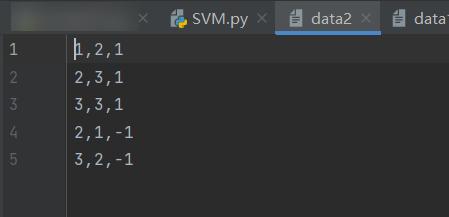提示，data2文件和代码文件要放在同一级目录下

学习总结：
支持向量机是很麻烦的一种统计方法，计算繁琐，所以可以使用代码来进行计算，相较于朴素贝叶斯、决策树这些算法，个人认为支持向量机很难
展开全文• ## python 实现支持向量机(SVM)

千次阅读 多人点赞 2019-02-25 16:38:13
现在我们回归正题，如果我们要求再高一点，假设决策面正好处于间隔区域的中轴线上，并且相应的支持向量对应的样本点到决策面的距离为d，那么公式⑦就可以进一步写成：    ⑧  公式两边同时除以d，得： ...

★ SVM简述：
(1.) 数据集线性可分：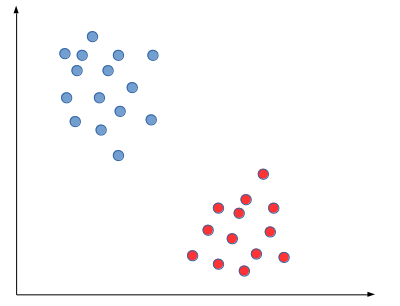对于线性可分的数据集，我们可以找到n个决策面将其分割开来，如下图所示：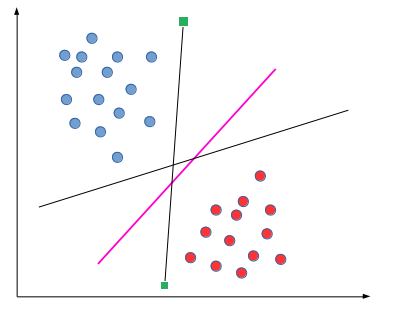由于我们的示例图是二维的，所以决策面在这里是一条直线，但是上图中的粉色线条的分类性能是优于其他线条的，这里涉及到第一个SVM独有的概念“分类间隔”——在保证决策面方向不变且不会出现错分样本的情况下移动决策面，会在原来的决策面两侧找到两个极限位置(越过该位置就会产生错分现象)，分类间隔越大，分类的容错率越高。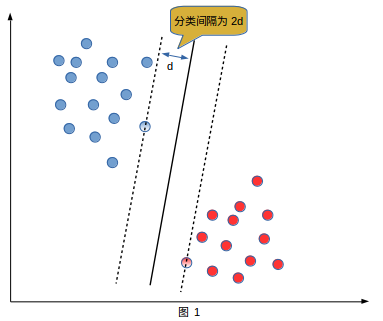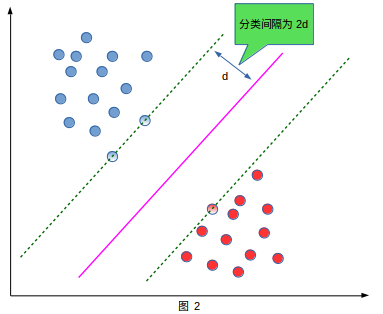a.    虚线的位置由决策面的方向和距离原决策面最近的几个样本的位置决定。
b.    这两条平行虚线正中间的分界线就是在保持当前决策面方向不变的前提下的最优决策面。
c.    两条虚线之间的垂直距离就是这个最优决策面对应的分类间隔。显然每一个可能把数据集正确分开的方向都有一个最优决策面(有些方向无论如何移动决策面的位置也不可能将两类样本完全分开)，而不同方向的最优决策面的分类间隔通常是不同的，那个具有“最大间隔”的决策面就是SVM要寻找的最优解。
d.    而这个真正的最优解对应的两侧虚线所穿过的样本点，就是SVM中的支持样本点，称为“支持向量”。
(2.) 数据集不是线性可分：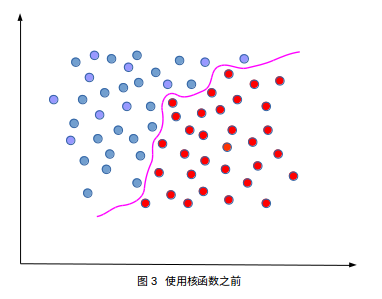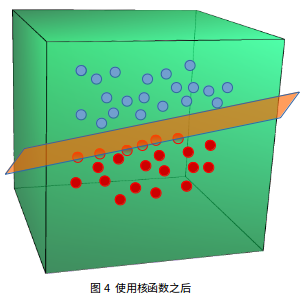当数据集不能用一条直线(或一个超平面)分割的时候，我们使用核函数将数据集映射到高维空间，然后在这高维空间里数据集会变得线性可分。

★ SVM问题剖析：
由上述可知，我们需要找到SVM的最大分类间隔。这就是我们的目标函数。
数据建模：
（1.) 公式表征：
二维空间下的一条直线可表示为：
①
现在我们做个小小的改变，让原来的x轴变成轴，y变成轴，于是公式①中的直线方程会变成下面的样子:
②
整理得:
③
写成向量乘积的形式：
④
用w表示上述列向量，x表示上述行向量，我们写成更一般的形式。从而有决策面方程：
⑤
其中：

在二维空间里，就是该直线的法向量，r 是直线的截距。其实，在n维空间中n-1维的超平面的方程形式也是公式⑤的样子，只不过向量 w，x 的维度从原来的2维变成了n维。
间隔的大小实际上就是支持向量对应的样本点到决策面的距离的二倍，如下图所示：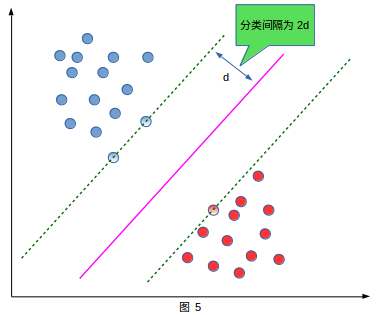我们已知了决策面方程(公式⑤)，由距离公式得出每个样本点到决策面的距离d为：
⑥
我们知道，分类间隔 interval= 2d，因此要想让分类间隔最大，就需要让d最大。
(2.) 约束条件：
首先我们给上述二维平面上的点进行分类标记：

如果我们的超平面方程能够完全正确地对上图的样本点进行分类，就会满足下面的方程：
⑦
假如对公式⑦有异议的，可设出该直线方程，再带入点，看看结果是否可归纳为公式⑦，也可稍加证明：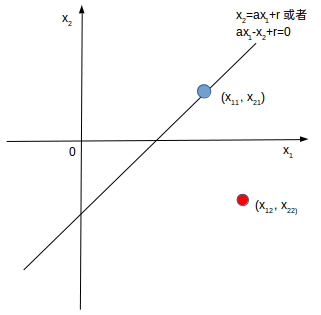证明：   设：

F(x1, x2)表示斜率为a(a>0)的任意直线。当它穿过蓝点(x11 , x21)时，由上图知：

从直线右边任取一点，如红点(x12, x22)，带入F(x1, x2)，得：

现在我们只需要判断c大于0或小于0就行了。我们将上述两个式子做差并整理：

由条件a>0，x12>x11，x22>x21知，c>0。证毕
这时a>0的情况，读者也可以用同样的方法证明a<0的情况，得到的结果是一样的。
现在我们回归正题，如果我们要求再高一点，假设决策面正好处于间隔区域的中轴线上，并且相应的支持向量对应的样本点到决策面的距离为d，那么公式⑦就可以进一步写成：
⑧
公式两边同时除以d，得：
⑨
其中：
⑩
把 和就当成一条直线的方向矢量和截距。你会发现事情没有发生任何变化，因为直线和直线其实是一条直线。现在，现在让我忘记原来的直线方程参数和，我们可以把参数和重新起个名字，就叫它们和。我们可以直接说：“对于存在分类间隔的两类样本点，我们一定可以找到一些决策面，使其对于所有的样本点均满足下面的条件：
⑪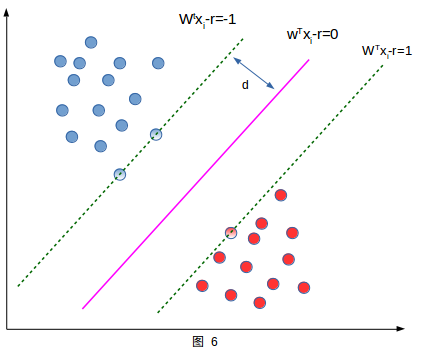(3.) 得到目标函数：
我们的目的是使分类间隔最大化，而在保证每一个样本点都正确分类的情况下，最大分类间隔就是支持向量与决策面距离的2倍，我们无需考虑其他样本点。因此距离d可表示为：
⑫
至于公式⑫的分子为何化为1，那是由公式⑧⑨⑪得到的。我们想最大化d，那么只需最小化||w||，即使最小，把类别标签和两个不等式⑪左边相乘，形成统一的表述：
⑬

★目标函数的优化
(一、)使用拉格朗日乘子法：(使用拉格郎日构造的目的就是把条件约束和目标函数放到一个式子，方便求最优)
我们得到的目标函数⑬是一个不等式约束条件下的优化问题。我们使用拉格朗日乘子法将目标函数f(x)和不等式约束条件h(x)构造成一个新的函数，然后在可行域里求得最优解。
在这里提一下拉格朗日乘子法的思路，它处理的约束条件分为两种，等式约束条件和不等式约束条件。我们设原始问题为min f(x)
(1.)等式约束条件： g(x)=0
x是向量，x取不同的值时，f(x)可看作映射在x构成的曲面(平面)上的n条等高线。而g(x)跟f(x)类似，可看作一条等高线。因此最优解就是f(x)与g(x)的切点。这里我们给出为什么最优点是切点而不是交点的理由：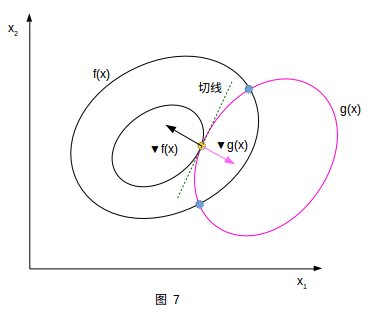如果最优解是交点，即梯度矢量不垂直于在点的切线方向，就会在的切线方向上存在不等于0的分量，也就是说在相遇点附近，还在沿着变化。这意味在上这一点的附近一定有一个点的函数值比更小，那么就不会是那个约束条件下的最优解了。所以，最优解是切点，并且梯度向量必然与约束条件的切线方向垂直。而函数的梯度方向必然与自身的等值线切线方向垂直，因此g(x)在最优点的梯度向量也与上述切线方向垂直，故，具有相同或相反的方向 。即：

为了体现数学的优美规范，我们还是构造拉格朗日函数：

我们将上式分别对x，求偏导，并令其为0，可得到求出最优解的解析式：
⑭
(2.)不等式约束条件：
类似等式约束条件，不过可行解区域不是一条线了，而是一个区域。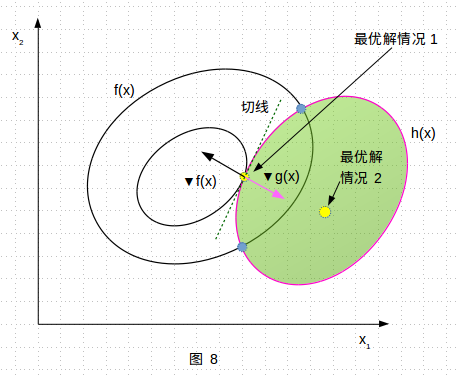最优解有两种情况，第一种情况，最优解在边界上，h(x)=0，目标函数 f(x) 在最优解附近的变化趋势是“在可行解区域内侧较大而在区域外侧较小”，与之对应的是函数 g(x) 在可行解区域内小于0，在区域外大于零，所以在最优解附近的变化趋势是内部较小而外部较大。这意味着目标函数 f(x) 的梯度方向与约束条件函数 g(x) 的梯度方向相反。因此由公式⑭知
第二种情况，最优解在可行解区域内部，h(x)<0，则相当于约束条件没有起作用，故拉格朗日函数中的参数。整合这两种情况，可以写出一个约束条件的统一表达(就是最优解必须满足下面的条件)，也被称为KKT条件：
⑮
(二、) 拉格郎日对偶:
对于约束条件下的极小值函数优化，我们可列出原始函数的解析式为：
⑯
我们由公式⑯构造广义拉格朗日函数：
⑰
其中是我们加入的系数变量，它可以根据我们的需要变大或变小。
再构造一个函数：
⑱
这里为什么要对取最大值，是因为只有取最大值才能还原我们的目标函数 f(x)。
我们把首先看作是的函数，x看作常数，求取最大值。
⑲
结合公式⑯的条件，我们对公式⑲进行简化，它分为两种情况：
(1.) 条件满足公式⑯，即在可行解区域内：(注意此时看作是的函数)
由于公式⑯:      ，所以公式⑲化简为：
⑳
(2.) 条件不满足公式⑯，即在可行解区域外：
Ⅰ.  若   即 (或 )，我们可以让 (或让)，则，公式⑲可化简为：
㉑
Ⅱ. 若，我们可以让，则，公式⑲可化简为：
㉑
综合(1.)和(2.)我们得到的表达式为：
㉒
现在约束条件已经没了，原始函数⑯化为：
㉓
尽管公式㉓描述的无约束优化问题看起来很美好，但我们很难建立的显示表达式，即很难直接从公式⑲里面把这两组参数拿掉，因为函数 在  为确定值时，是  的线性函数，其极大值并不在梯度为0的地方,这样我们就没法通过令的方法求解出最优解。为此我们需要找到公式㉓的对偶问题。我们再构造另一个函数，表达式为：
㉔
另外一个优化问题的描述是：
㉕
公式㉓ 、㉕就是一组对偶问题，如果我们能够想办法证明㉓和㉕存在相同的解，那我们就可以在对偶问题中选择比较简单的一个来求解。
✿ 对偶问题同解的证明:
㉖
此公式㉖可由公式⑯⑰推出来(因为 && ，故 )
由公式㉖得：
㉗
这里的分别是对偶问题和原始问题的最优值。公式㉗称为弱对偶，即对所有优化问题都成立。与之对应的还有强对偶，，我们介绍一个定理：
对于原始问题和对偶问题，假设函数和不等式约束条件为凸函数，等式约束条件中的为仿射函数(即由一阶多项式构成的函数，，均为列向量，为标量)；并且至少存在一个使所有不等式约束条件严格成立，即，则存在使得是原始问题的最优解，是对偶问题的最优解且有：，并其充分必要条件如下：
㉘
该定理的证明网址是：https://web.stanford.edu/~boyd/cvxbook/bv_cvxbook.pdf
(三、)总结一下约束条件下的函数优化问题的步骤(使用拉格朗日乘子法和拉格朗日对偶)：
1.  写出原始问题的对偶问题的表达式
2.  把对偶表达式对x求导并令其为0，此时看作常数。
3.   得到的x的表达式是的函数，再对分别求偏导令其为0，得出的值。
4.   把第3步得到的的值带入到步骤2中的x表达式，得出x的值。
✿  举个例子：
一个优化问题的数学描述为：

构造广义拉格朗日函数为(注意看上式，就是一个等式约束和一个不等式约束)：

其对偶问题为：

我们将拉格朗日函数分别对求偏导并令其为0，得：

整理得：

将其带入到拉格朗日函数，得到：

将上式分别对求偏导并令其为0，得：

从而解出：

★在支持向量机中使用拉格郎日对偶：
我们的原始问题是：

明显是一个有多个不等式约束条件的优化问题，构造广义拉格郎日函数：
㉙
这里，，m是样本点个数。我们需要找到原始问题的对偶问题：
㉚
我们把分别对 求偏导，并令其为0，得：

㉛
说明一下：在公式㉚中：
，       ，
所以整理公式㉛，得出结果：
㉜
把公式㉜带入到公式㉚中，得：
㉝
根据乘法的基本规律(本质就是一个列向量转置然后点乘它本身)：

所以公式㉝化简为：
㉞
因此原始函数的对偶函数为：
㉟

★ 引入松弛变量和惩罚因子：
(一、) 引入原因：
我们的数据集并不总是线性可分，有可能存在个别离群点(有可能是噪声造成的)使我们找不到一个决策面将数据集严格分类。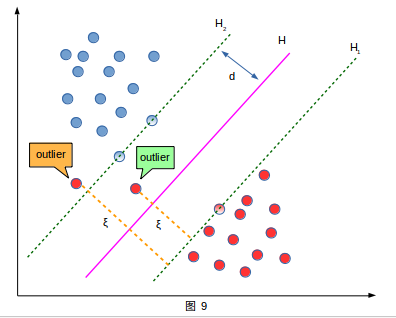上图中存在两个异常点，我们称之为outlier。
黄色虚线是这个离群点距离它原本的边缘所超出的权重距离，我们称之为该outlier对应的松弛变量。
我们回顾公式⑪：
⑪

该公式意思是：在每个样本点被正确分类的前提下，样本点到决策面的距离大于等于1，作为支持向量的样本点到决策面的距离等于1，现在我们引入松弛变量，

因为松弛变量是非负的，因此最终结果是要求间隔可以比1小，某些点出现这种间隔小于1的情况(outlier)，意味着我们放弃了对这些点的精确分类，这对分类器是一种损失，我们的目标是让最小，因而这种损失必然是使之增大，不然就不叫损失了，如何衡量这种损失，常用的方式有两种：
<1.>                                       <2.>
我们把损失加入到目标函数里，就需要一个惩罚因子，，因此优化问题就变成：
㊱
✿ 注意：
(1.) 不是所有样本点都有与之对应的松弛变量，只有离群点才有，或者说被分类正确的样本点的松弛变量为0。
(2.) 太小，分离超平面就计算不出来了，或者只能计算一个不佳的决策面 ；如果太大了，那任何一个超平面都是分类器。
(3.) 惩罚因子C决定了你有多重视离群点带来的损失，当所有离群点松弛变量的和一定时，给定的C越大，对目标函数的损失越大，这就意味着你非常不愿意放弃这些离群点，它就按“最正确”的来，容易过拟合；C越小，分类器越不在乎错误，就是欠拟合。
(4.) 惩罚因子是你给定的一个值，但没有规定说所有松弛变量都使用一个惩罚因子C，完全可以给每一个离群点设置不同的C。
根据上述优化问题我们还是老套路写出它的广义拉格郎日函数：
㊲
原始问题为：

对偶问题为:

我们将拉格朗日函数分别对求偏导，即对公式㊲求偏导得：
㊳
把公式㊳带入到对偶问题中，得到：
㊴
即对偶函数为：
㊵
✿ 我们分析一下样本点的取值情况：
首先我们针对原始问题的最优解罗列KKT条件：

分类讨论的取值情况：
<1.> 时：
因为>0，所以，因为，所以，又有，所以，所以
<2.> 时：
因为，所以，又有，所以，因为，所以，因此。这里我认为是严格小于，这是从推导KKT条件的过程中得到的，有些博客中是小于等于。
<3.> 时：
因为，所以，又有，所以，因为，所以，因此
综合上述知：在最优点处(找到最优，即最优决策面)，所有样本点满足：
㊶
✿ 通俗解释：
当时，样本点为支持向量；
当时，样本点为除支持向量以外的正确分类的点；
当时，样本点为未被正确分类的点(离群点）。

★ SMO算法
上一章我们已经得到对偶函数的表达式，我们只需解出，然后根据公式㊳就能解出，从而得到最优决策面。如何解出就需要用到SMO算法了。
(一、) SMO算法公式推导：
我们待优化问题是：

SMO算法的基本思路是：
，每次选择两个个变量，固定其他参数。假设选择优化的是，那么需要固定其他m-2个参数。因此目标函数可写为：
㊷
其中：

Constant代表与无关的项，因为，所以公式㊷可以简化为：
㊸
由于约束条件    和 ，所以：
㊹
可见为定值，对公式㊹两边同时乘以，又有公式  ，得：
㊺
把公式㊺带入到公式㊸中，得：
㊻
我们将公式㊻对求导并令其为0 ( ☂为啥这么做呢，你看公式㊻像不像关于的二次函数，那么求导并令其为0不就是极值，这里我们假设，这样二次函数才是开口向上，才是极小值点，其实也好证明，，因为，所以，但它也有例外，那就是对于不满足Mercer定理的核函数，该证明不成立)，令公式㊻为，得：
㊼
假如我们通过上式㊼求得了，再带入公式㊺即可求得，我们分别标记为，而优化之前，我们记为，有公式㊹知：
㊽
我们上面已经求得： ，所以SVM的超平面为：
㊾
又有:

则：
㊿
我们把公式㊽、㊿带入到公式㊼中，，整理：
<51>
✿ 有的朋友在这里的公式推导出现了问题，我们来推导一下公式<51>的由来：
观察公式㊼，先计算 ，得：

因为由公式㊺知：  ，且 ， 带入得：

因为 ，带入得：

注意此时的是未优化之前的，故应该写成，得：

将此结果带入公式㊼，替换掉公式㊼的，得：
<51>
☂  注意： 虽然我们算出来了的表达式，但是这个表达式的推导过程没有考虑  这个约束条件，我们需要根据  和  求出的取值范围，如果在范围内，我们应用公式<51.>来更新，如果在取值范围外，我们需要用最小值和最大值来更新它。因此我们需要求出的可行域。(再通俗一点的解释就是：刚才我们求导令其为0才得到的极值点，但是我们没考虑定义域啊，极值点在定义域内确实可以根据这个公式得到最小值点，要是极值点在定义域左边呢，在定义域右边呢，这就需要讨论了)
✿  根据约束条件我们画出的可行域(因为固定了除以外的其他参数，所以约束条件改成下式了)：

在二维平面上表达两个约束条件：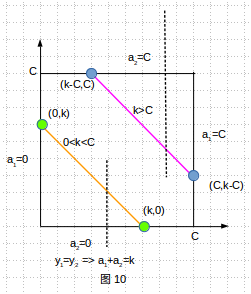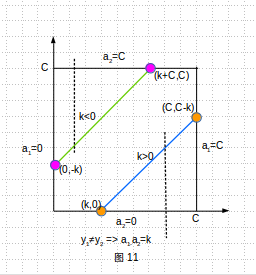说明：图中方形区域表示的是 和  共同围起来的区域，几条直线对应的是几种不同的的情况，因为 和  只能取值为+1或-1，所以按照 和  的取值情况，可分为下面四种情况：
(1.)  当 时，此时表达式是，对应左图(图10)，根据的取值情况，又可分为四种情况来求解的可行域。
❶ 当 时，因为，所以此时可行域为空。并且也不会出现  的情况，因为，所以
❷ 当 时，对应左图(图10)中的橘黄色直线，可以这样分析：因为，可以把看作截距，截距在(0,C)上变化，我们要看的可行域可以参考初中的方法，垂直于轴画一条虚线(如图中黑色虚线)，则的可行域为，又有，所以 ，取最大的，即的可行域为[0，]，即[0，]
❸ 当 时，对应左图(图10)中的粉红色直线，根据❷相同的方法，则的可行域为，又有，所以 ，取最小的，即的可行域为[-C，C]，即[，C]
❹ 当 时，因为，又有且，两个不等式相加得：，与假设矛盾，所以此时可行域为空。即不存在此情况。
综合上述，在情况(1.)下，我们得到的可行域是L=max(0，)； H=min(，C)
(2.) 当 时，此时表达式是，对应左图(图10)，我们用k替换，得到是：不就和(1.)一样的分析方法了，只是把(1.)的所有换成k。
(3.) 当且 时，此时表达式是 ，即，对应右图(图11)，根据的取值情况，又可分为四种情况来求解的可行域。
❶ 当时，因为且，相加得：，条件与之矛盾，所以不存在此情况。
❷ 当 时，对应右图(图11)中的绿色直线，用上述相同的方法，我们得到的可行域为，又有，所以 ，取最小的，即的可行域为[ ，C]，即[，C]
❸ 当 时，对应右图(图11)中的蓝色直线，我们得到的可行域为，又有，所以 ，取最大的，即的可行域为[0，]，即[0，]
❹ 当 时，因为且，相加得：，条件与之矛盾，所以不存在此情况。
综合上述，在情况(3.)下，我们得到的可行域是L=max(0，)； H=min(，C)
(4.) 当且 时，此时表达式是 ，对应右图(图11)，我们用k替换，得到是： 就和(3.)一样的分析方法了，只是把(3.)的所有换成k。
☂ 综合上述四种情况，我们得出：
<52.>
✿ 前面求出了在可行域内的的更新公式，刚刚我们又得到了的最大和最小值，整合到一个表达式为：
<53.>
假如我们真遇到了不满足Mercer定理的核函数，那么会存在或者，当表达式为0的时候，是条直线，最小值就是边界值，算算两个边界值，哪个小就用哪个；假如表达式大于0，是个开口向下的二次函数，最小值也在边界取得。
求出后，我们便可以通过公式㊽求出，即：
㊽
(二、) 的选择问题：
回顾以前：在最优点处(找到最优，即最优决策面)，所有样本点满足：
㊶
根据上述讨论，我们知道只要得到的值，我们就能通过公式计算出，从而得到最优决策面，这就意味着我们需要让所有样本点对应的满足KKT条件(即公式㊶)。
✿ 编程思想：
1.  首先我们初始化  为0，即开始时每个样本点对应的  为0。我们的决策面方程的截距  也初始化为0
2.  每次我们选取两个拉格朗日乘子进行优化，选择第一个乘子是外循环，外循环寻找有两种遍历方式：一种是按样本点的本身顺序遍历所有的样本点，寻找不满足KKT条件的，这里不满足KKT条件是指违反公式㊶，违反公式有三种情况：

❤ 小提示： 我们的目的就是优化这些违反KKT的乘子 使其满足KKT条件。对于第一条不满足KKT的乘子，本应该，但是它却，所以我们需要减小，这里设置违反条件时，根据公式㊶，它的互补条件应该是，而这里我只写了，这是因为我们以后会对进行区间化处理， 的情况明显不符合每个乘子的规定，所以 在这里我们不需要考虑。而且我们优先选择前两条的违反KKT的乘子，因为第三条为了让乘子满足KKT条件  ，需要让 变大 或者让减小，而乘子的更新公式为：，我们知道 (除非应用了不满足Mercer定理的核函数)，当时，要想让它变大，就需要让 成立，而很多时候我们不能保证它的成立，假如不成立即时，由于本身的限制，所以此时不会改变，更新失败；当时，也是这么分析。这就是一般博客会说的边界上()的值一般不会轻易改变。因此我们选择第一个乘子的筛选条件就是：，但是或者太过苛刻，我们可以设置一个容许误差tol，tol一般取 ，所以筛选条件变为：。筛选出第一个不满足KKT条件的乘子之后，我们把该朗格朗日乘子对应的Ei也算出来，并保存起来，然后我们接着选择第二个乘子，第二个乘子我们以最大化优化步长为目标来选择，第二个乘子的优化步长大致正比于，因此选最大化的，这从哪些样本点中选择呢，我们从已经计算好Ei的且的样本点中选择，这些计算好的Ei的样本点就是我们按顺序扫描过的且不满足KKT的样本点。
3.  第二种寻找第一个乘子的方式是：遍历非边界(即满足)，令其满足KKT条件。
4.  我们做法是先使用第一种方式对全部样本点进行单遍扫描，找出的样本继续用第二种扫描方式进行多遍扫描，直至的样本满足KKT条件(即不再改变)，此时再进行方式一来扫描，如果还是没有改变，那么退出循环。
✿ 对决策面截距的更新：
有m个，但是只有一个，每一次更新完之后，都需要更新，如果某个更新完以后是一个支持向量的参数，即，那么此时根据这个计算出来的是比较准确的，而支持向量满足：，又有，所以：
<54.>
因此：
<55.>
<56.>
将公式<55.>和公式<56.>相加，整理得：
<57.>
我们也可以写出:

在训练时，满足：
<58.>

★ 代码：
from numpy import *
import random
import matplotlib.pyplot as plt
import numpy

def kernelTrans(X,A,kTup):                    # 核函数(此例未使用)
m,n=shape(X)
K = mat(zeros((m,1)))
if kTup =='lin':
K=X*A.T
elif kTup=='rbf':
for j in range(m):
deltaRow = X[j,:]-A
K[j]=deltaRow*deltaRow.T           # ||w||^2 = w^T * w
K =exp(K/(-1*kTup**2))              # K = e^(||x-y||^2 / (-2*sigma^2))
else:
raise NameError("Houston we Have a problem --")
return K

class optStruct:
def __init__(self,dataMain,classLabel,C,toler,kTup):

self.X = dataMain                     # 样本矩阵
self.labelMat = classLabel
self.C = C                            # 惩罚因子
self.tol = toler                      # 容错率
self.m = shape(dataMain)           # 样本点个数
self.alphas = mat(zeros((self.m,1)))  # 产生m个拉格郎日乘子，组成一个m×1的矩阵
self.b =0                             # 决策面的截距
self.eCache = mat(zeros((self.m,2)))    # 产生m个误差 E=f(x)-y ,设置成m×2的矩阵，矩阵第一列是标志位，标志为1就是E计算好了，第二列是误差E
# self.K = mat(zeros((self.m,self.m)))
# for i in range(self.m):               # K[,]保存的是任意样本之间的相似度(用高斯核函数表示的相似度)
#     self.K[:,i]=kernelTrans(self.X,self.X[i,:],kTup)

dataMat = []
labelMat = []
fr = open(filename)
lineArr = line.split()
dataMat.append([float(lineArr),float(lineArr)])
labelMat.append(float(lineArr))     # 一维列表
return dataMat, labelMat

def selectJrand(i, m):       # 随机选择一个不等于i的下标
j =i
while(j==i):
j = int(random.uniform(0,m))
return j

def clipAlpha(aj, H,L):
if aj>H:                      # 如果a^new 大于上限值，那么就把上限赋给它
aj = H
if L>aj:                      # 如果a^new 小于下限值，那么就把下限赋给它
aj = L
return aj

def calcEk(oS, k):           # 计算误差E, k代表第k个样本点，它是下标，oS是optStruct类的实例
# fXk = float(multiply(oS.alphas,oS.labelMat).T * oS.K[:,k] + oS.b)   # 公式f(x)=sum(ai*yi*xi^T*x)+b
fXk = float(multiply(oS.alphas,oS.labelMat).T * (oS.X*oS.X[k,:].T)) +oS.b
Ek = fXk - float(oS.labelMat[k])          # 计算误差 E=f(x)-y
return Ek

def selectJ(i, oS, Ei):      # 选择两个拉格郎日乘子,在所有样本点的误差计算完毕之后，寻找误差变化最大的那个样本点及其误差
maxK = -1                # 最大步长的因子的下标
maxDeltaE = 0            # 最大步长
Ej = 0                   # 最大步长的因子的误差
oS.eCache[i] = [1,Ei]
valiEcacheList = nonzero(oS.eCache[:,0].A)    # nonzero结果是两个array数组，第一个数组是不为0的元素的x坐标，第二个数组是该位置的y坐标
# 此处寻找误差矩阵第一列不为0的数的下标
print("valiEcacheList is {}".format(valiEcacheList))
if (len(valiEcacheList))>1:
for k in valiEcacheList:          # 遍历所有计算好的Ei的下标，valiEcacheLIst保存了所有样本点的E，计算好的有效位置是1,没计算好的是0

if k == i:
continue
Ek = calcEk(oS,k)
deltaE = abs(Ei-Ek)          # 距离第一个拉格朗日乘子a1绝对值最远的作为第二个朗格朗日乘子a2
if deltaE>maxDeltaE:
maxK = k                 # 记录选中的这个乘子a2的下标
maxDeltaE = deltaE       # 记录他俩的绝对值
Ej = Ek                  # 记录a2此时的误差
return maxK, Ej
else:                             # 如果是第一次循环，随机选择一个alphas
j = selectJrand(i, oS.m)
# j = 72
Ej = calcEk(oS, j)
return j,Ej

def updateEk(oS, k):
Ek = calcEk(oS, k)
oS.eCache[k] = [1,Ek]        # 把第k个样本点的误差计算出来，并存入误差矩阵，有效位置设为1

def innerL(i, oS):
Ei = calcEk(oS, i)           # KKT条件， 若yi*(w^T * x +b)-1<0 则 ai=C  若yi*(w^T * x +b)-1>0 则 ai=0
print("i is {0},Ei is {1}".format(i,Ei))
if ((oS.labelMat[i]*Ei < -oS.tol) and (oS.alphas[i] < oS.C)) or ((oS.labelMat[i]*Ei > oS.tol) and (oS.alphas[i] > 0)):
j,Ej = selectJ(i,oS,Ei)
print("第二个因子的坐标{}".format(j))
alphaIold = oS.alphas[i].copy()       # 用了浅拷贝， alphaIold 就是old a1,对应公式
alphaJold = oS.alphas[j].copy()
if oS.labelMat[i] != oS.labelMat[j]:  # 也是根据公式来的，y1 不等于 y2时
L = max(0,oS.alphas[j] - oS.alphas[i])
H = min(oS.C, oS.C+oS.alphas[j]-oS.alphas[i])
else:
L = max(0,oS.alphas[j]+oS.alphas[i]-oS.C)
H = min(oS.C,oS.alphas[j]+oS.alphas[i])
if L==H:         # 如果这个j让L=H,i和j这两个样本是同一类别，且ai=aj=0或ai=aj=C，或者不同类别，aj=C且ai=0
# 当同类别时 ai+aj = 常数 ai是不满足KKT的，假设ai=0,需增大它，那么就得减少aj，aj已经是0了，不能最小了，所以此情况不允许发生
# 当不同类别时 ai-aj=常数，ai是不满足KKT的，ai=0,aj=C,ai需增大，它则aj也会变大，但是aj已经是C的不能再大了，故此情况不允许
print("L=H")
return 0
# eta = 2.0*oS.K[i,j]-oS.K[i,i]-oS.K[j,j]   # eta=K11+K22-2*K12
eta = 2.0*oS.X[i,:]*oS.X[j,:].T - oS.X[i,:]*oS.X[i,:].T - oS.X[j,:]*oS.X[j,:].T
if eta >= 0:                 # 这里跟公式正好差了一个负号，所以对应公式里的 K11+K22-2*K12 <=0，即开口向下，或为0成一条直线的情况不考虑
print("eta>=0")
return 0
oS.alphas[j]-=oS.labelMat[j]*(Ei-Ej)/eta     # a2^new = a2^old+y2(E1-E2)/eta
print("a2 归约之前是{}".format(oS.alphas[j]))
oS.alphas[j]=clipAlpha(oS.alphas[j],H,L)     # 根据公式，看看得到的a2^new是否在上下限之内
print("a2 归约之后is {}".format(oS.alphas[j]))
# updateEk(oS,j)               # 把更新后的a2^new的E更新一下
if abs(oS.alphas[j]-alphaJold)<0.00001:
print("j not moving enough")
return 0
oS.alphas[i] +=oS.labelMat[j]*oS.labelMat[i]*(alphaJold-oS.alphas[j])   # 根据公式a1^new = a1^old+y1*y2*(a2^old-a2^new)
print("a1更新之后是{}".format(oS.alphas[i]))
# updateEk(oS,i)
# b1^new = b1^old+(a1^old-a1^new)y1*K11+(a2^old-a2^new)y2*K12-E1
# b1 = oS.b-Ei-oS.labelMat[i]*(oS.alphas[i]-alphaIold)*oS.K[i,i]-oS.labelMat[j]*\
#      (oS.alphas[j]-alphaJold)*oS.K[i,j]

b1 = oS.b-Ei-oS.labelMat[i]*(oS.alphas[i]-alphaIold)*oS.X[i,:]*oS.X[i,:].T-oS.labelMat[j]* \
(oS.alphas[j]-alphaJold)*oS.X[i,:]*oS.X[j,:].T
# b2 = oS.b-Ej-oS.labelMat[i]*(oS.alphas[i]-alphaIold)*oS.K[i,j]-oS.labelMat[j]* \
#      (oS.alphas[j]-alphaJold)*oS.K[j,j]

b2 = oS.b-Ej-oS.labelMat[i]*(oS.alphas[i]-alphaIold)*oS.X[i,:]*oS.X[j,:].T-oS.labelMat[j]* \
(oS.alphas[j]-alphaJold)*oS.X[j,:]*oS.X[j,:].T
updateEk(oS,j)          # 个人认为更新误差应在更新b之后，因为公式算出的b的公式使用的是以前的Ei
updateEk(oS,i)
# b2^new=b2^old+(a1^old-a1^new)y1*K12+(a2^old-a2^new)y2*K22-E2
if (0 < oS.alphas[i]) and (oS.C > oS.alphas[i]):
oS.b = b1.A
elif (0<oS.alphas[j]) and (oS.C > oS.alphas[j]):
oS.b = b2.A
else:
oS.b = (b1+b2)/2.0

print("b is {}".format(oS.b))
return 1
else:
return 0

def smoP(dataMatIn, classLabels, C,toler,maxIter,kTup=('lin',)):
oS = optStruct(mat(dataMatIn), mat(classLabels).transpose(),C,toler,kTup)
iter = 0
entireSet = True              # 两种遍历方式交替
alphaPairsChanged = 0
while (iter<maxIter) and ((alphaPairsChanged>0) or (entireSet)):
alphaPairsChanged = 0
if entireSet:
for i in range(oS.m):
alphaPairsChanged += innerL(i,oS)
print("fullSet, iter:%d i: %d pairs changed %d"%(iter,i ,alphaPairsChanged))

iter+=1
print("第一种遍历alphaRairChanged is {}".format(alphaPairsChanged))
print("-----------eCache is {}".format(oS.eCache))
print("***********alphas is {}".format(oS.alphas))
print("---------------------------------------")
else:
nonBoundIs = nonzero((oS.alphas.A > 0) * (oS.alphas.A < C))  # 这时数组相乘，里面其实是True 和False的数组，得出来的是
# 大于0并且小于C的alpha的下标
for i in nonBoundIs:
alphaPairsChanged += innerL(i,oS)
print("non-bound, iter: %d i:%d, pairs changed %d"%(iter,i,alphaPairsChanged))
print("第二种遍历alphaPairChanged is {}".format(alphaPairsChanged))
iter+=1
if entireSet:
entireSet = False  # 当第二种遍历方式alpha不再变化，那么继续第一种方式扫描，第一种方式不再变化，此时alphachanged为0且entireSet为false，退出循环
elif (alphaPairsChanged==0):
entireSet=True
print("iteration number: %d"%iter)
return oS.b,oS.alphas

def calcWs(alphas,dataArr,classLabels):                # 通过alpha来计算w
X = mat(dataArr)
labelMat = mat(classLabels).transpose()
m,n = shape(X)
w = zeros((n,1))
for i in range(m):
w += multiply(alphas[i]*labelMat[i], X[i,:].T)        # w = sum(ai*yi*xi)
return w

def draw_points(dataArr,classlabel, w,b,alphas):
myfont = FontProperties(fname='/usr/share/fonts/simhei.ttf')    # 显示中文
plt.rcParams['axes.unicode_minus'] = False     # 防止坐标轴的‘-’变为方块
m = len(classlabel)
red_points_x=[]
red_points_y =[]
blue_points_x=[]
blue_points_y =[]
svc_points_x =[]
svc_points_y =[]
# print(type(alphas))
svc_point_index = nonzero((alphas.A>0) * (alphas.A <0.8))
svc_points = array(dataArr)[svc_point_index]
svc_points_x = [x for x in list(svc_points)]
svc_points_y = [x for x in list(svc_points)]
print("svc_points_x",svc_points_x)
print("svc_points_y",svc_points_y)

for i in range(m):
if classlabel[i] ==1:
red_points_x.append(dataArr[i])
red_points_y.append(dataArr[i])
else:
blue_points_x.append(dataArr[i])
blue_points_y.append(dataArr[i])

fig = plt.figure()                     # 创建画布
ax.set_title("SVM-Classify")           # 设置图片标题
ax.set_xlabel("x")                     # 设置坐标名称
ax.set_ylabel("y")
ax1=ax.scatter(red_points_x, red_points_y, s=30,c='red', marker='s')   #s是shape大小，c是颜色，marker是形状，'s'代表是正方形,默认'o'是圆圈
ax2=ax.scatter(blue_points_x, blue_points_y, s=40,c='green')
# ax.set_ylim([-6,5])
print("b",b)
print("w",w)
x = arange(-4.0, 4.0, 0.1)                   # 分界线x范围,步长为0.1
# x = arange(-2.0,10.0)
if isinstance(b,numpy.matrixlib.defmatrix.matrix):
b = b.A
y = (-b-w*x)/w    # 直线方程 Ax + By + C = 0
ax3,=plt.plot(x,y, 'k')
ax4=plt.scatter(svc_points_x,svc_points_y,s=50,c='orange',marker='p')
plt.legend([ax1, ax2,ax3,ax4], ["red points","blue points", "decision boundary","support vector"], loc='lower right')         # 标注
plt.show()

b,alphas = smoP(dataArr,labelArr,0.8,0.001,40)
w=calcWs(alphas,dataArr,labelArr)
draw_points(dataArr,labelArr,w,b,alphas)
★ 运行结果：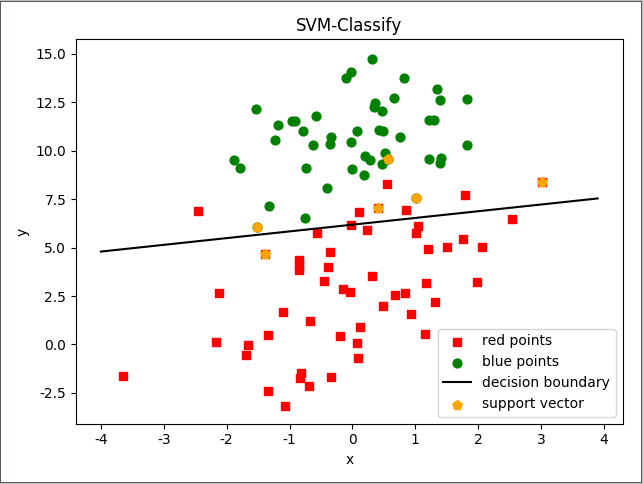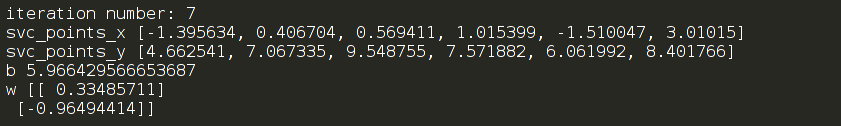★ 数据集：
-0.397822   8.058397    -1 0.824839    13.730343   -1 1.507278    5.027866    1 0.099671    6.835839    1 -0.344008   10.717485   -1 1.785928    7.718645    1 -0.918801   11.560217   -1 -0.364009   4.747300    1 -0.841722   4.119083    1 0.490426    1.960539    1 -0.007194   9.075792    -1 0.356107    12.447863   -1 0.342578    12.281162   -1 -0.810823   -1.466018   1 2.530777    6.476801    1 1.296683    11.607559   -1 0.475487    12.040035   -1 -0.783277   11.009725   -1 0.074798    11.023650   -1 -1.337472   0.468339    1 -0.102781   13.763651   -1 -0.147324   2.874846    1 0.518389    9.887035    -1 1.015399    7.571882    -1 -1.658086   -0.027255   1 1.319944    2.171228    1 2.056216    5.019981    1 -0.851633   4.375691    1 -1.510047   6.061992    -1 -1.076637   -3.181888   1 1.821096    10.283990   -1 3.010150    8.401766    1 -1.099458   1.688274    1 -0.834872   -1.733869   1 -0.846637   3.849075    1 1.400102    12.628781   -1 1.752842    5.468166    1 0.078557    0.059736    1 0.089392    -0.715300   1 1.825662    12.693808   -1 0.197445    9.744638    -1 0.126117    0.922311    1 -0.679797   1.220530    1 0.677983    2.556666    1 0.761349    10.693862   -1 -2.168791   0.143632    1 1.388610    9.341997    -1 0.317029    14.739025   -1

★ 参考链接有：
1. https://zhuanlan.zhihu.com/p/29865057
2. https://www.cnblogs.com/xxrxxr/p/7538430.html#commentform
3. https://blog.csdn.net/lx_ros/article/details/80772130
4. https://blog.csdn.net/siyue0211/article/details/80607566
5. https://blog.csdn.net/qll125596718/article/details/6910921
6. https://blog.csdn.net/v_july_v/article/details/7624837#commentBox
8. https://wenku.baidu.com/view/aeba21be960590c69ec3769e.html

展开全文监督学习 算法 机器学习...

# 支持向量机pythonpython 订阅